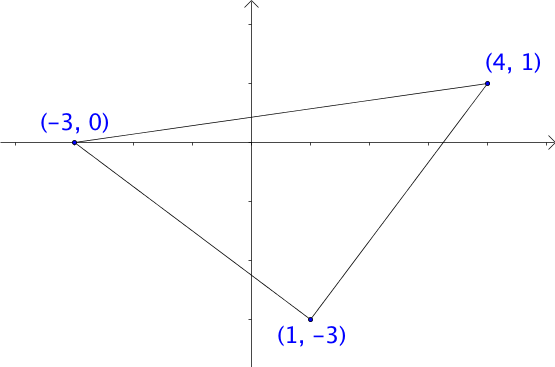# Practice: Find The Perimeter Of A TriangleLet there be a triangle drawn on a Cartesian plane with vertices at coordinates $(-3,0), (1,-3), (4,1).$ Find the perimeter of this triangle to the nearest integer.

×